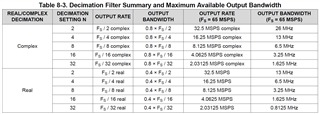If you have a related question, please click the "Ask a related question" button in the top right corner. The newly created question will be automatically linked to this question.

# ADC3660: Out put rate at the customer conditions

Hi Team,

I have 2 questions as follows.

[Q1]
The customer would like to use the following specifications.
- Decimation: 32
- Output mode: Real
- Output resolution: 20bit
- Output interface: 2-Wire

Is the OUTPUT RATE in this conditions 2.03125 MSPS as shown in the data sheet Table 8-3?

[Q2]
Does the OUTPUT RATE in Table 8-3 change depending on the output resolution and interface?
If yes, please tell me all the OUTPUT RATE at 14, 16, 18 and 20 bits.Best Regards,

• Hi Atsushi,

Yes, the 2.03125MSPS output data rate is correct. The output data rate will remain the same regardless of resolution and interface modes. This is because the output data rate is dependent only upon sample rate and decimation mode. In any of these decimation modes, the calculations are performed at a 20 bit resolution to avoid degrading the sampled data, and the result is then truncated back to the selected resolution (14,16,18,20). I am wondering if you are meaning to ask about the serialization rate instead. The serialization rate will change based on the selected ADC resolution. More info on this is on Table 8-7 in the datasheet, in the section Output Formatter.

Regards, Chase

• Hi Atsushi,

To clarify, the datasheet states that "the actual data output rate depends on the output resolution and number of lanes used". The terminology "actual data output rate" is the same as the "serialization rate" as Chase mentions above.

Regards, Amy

I'd like to know about the actual data output rate mentioned below.

8.3.5 Digital Interface
"The actual data output rate depends on the output resolution and number of lanes used."

What is the actual output data rate for 20-bit resolution and 2-wire?
What formula is used to calculate 2.03125MSPS in Table 8-3?

Best Regards,

• I'm sorry for my error operation.

This case is not closed.

• Hi Atsushi,

As Amy mentioned, the "actual output data rate" is referring to the serialization rate. The "actual output data rate" is as follows:
20 bit, 2-wire mode, the serialization factor is 10x. This means the data clock will be Fs*5. At each data clock transition, there are 2 samples of data (per each channel) in 2 wire mode. I've modified figure 8-35 to show how this will look for 10x serialization mode. There will be 8 bits per DCLK period, meaning the "actual output data rate" is DCLK * 8 bits. If the sample clock is 65 MSPS, then DCLK will be 650 MSPS, meaning the actual output data rate is 5.2Gbps -> 2.6 Gbps per ADC -> each lane at 1.3GbpsThe 2.03125 MSPS is calculated as Fs / decimation rate.

Regards, Chase